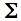Linear Correlation and Regression
Data-ImportVersion
For a sample of N bivariate values of X and Y, this page will calculate:T
 r = the Pearson product-moment correlation coefficient;T r2 = the coefficient of determination;T the slope of the regression line;T the Y intercept of the regression line;T the standard error of estimate;T the value of t associated with the calculated value of r, along with the corresponding one- and two-tailed probabilities;T the residual for each value of Y, calculated as residual = Y—(intercept+[slope(X)])T the lower and upper limits of the .95 and .99 confidence intervals for the correlation coefficient (rho) that exists within the bivariate population from which the sample is drawn;     andT the lower and upper limits of the .95 and .99 confidence intervals for the slope of the regression.
 The logic and computational details of correlation and regression are described in Chapter 3 of Concepts and Applications.
• Importing Data via Copy & Paste:T
When importing data from a spreadsheet, the paired values of X and Y must be in the form of two adjacent columns. Within the spreadsheet application, select and copy the two columns of data. Then return to this page, click the cursor into the data-entry field and perform the 'Paste' operation.T
• Direct Entry into Data Field:T
Click the cursor into the empty data-entry field, enter the first value of X; then press the space bar one (and only one) time; then enter the value of Y; then press the carriage return key. Do the same for subsequent paired values of X and Y, except that the final entry should not be followed by a carriage return.T
• Data Check:T
Make sure that the final line in the data-entry field entry is not followed by a carriage return. (An extra carriage return at the end of the list will be interpreted as an extra data entry whose value is zero. Importing data via the copy and paste procedure will almost always produce an extra carriage return at the end of a column.) After the bivariate list has been pasted into the data-entry field, click the cursor immediately to the right of the final entry in the list, then press the down-arrow key. If an extra line is present, the cursor will move downward. Extra lines can be removed by pressing the down arrow key until the cursor no longer moves, and then pressing the 'Backspace' key (on a Mac platform, 'delete') until the cursor stands immediately to the right of the final entry.T
• After verification of data entry, click the button labeled «Calculate».
 Data Entry Data Report Please remember toperform the Data Checkprocedure. Column 1: X Column 2: Y Column 3: Residual
Data SummaryT
 iX = iX2i = iY = iY2i = iXY =
 X Y N Mean Variance Std.Dev. Std.Err.
 r r2 Slope YIntercept Std. Err. ofEstimate
 t df
 P one-tailed two-tailed

0.95 and 0.99 Confidence Intervals for rhoQ
 Lower Limit Upper Limit 0.95 0.99

0.95 and 0.99 Confidence Intervals for the Slope of the RegressionQ
 Lower Limit Upper Limit 0.95 0.99

 Home Click this link only if you did not arrive here via the VassarStats main page.